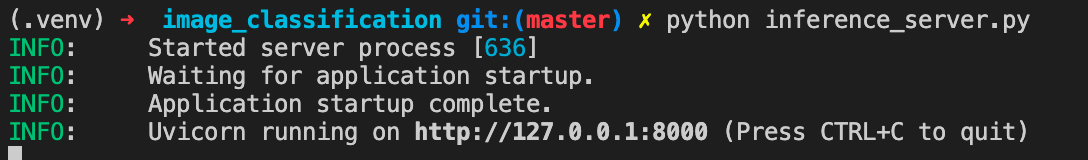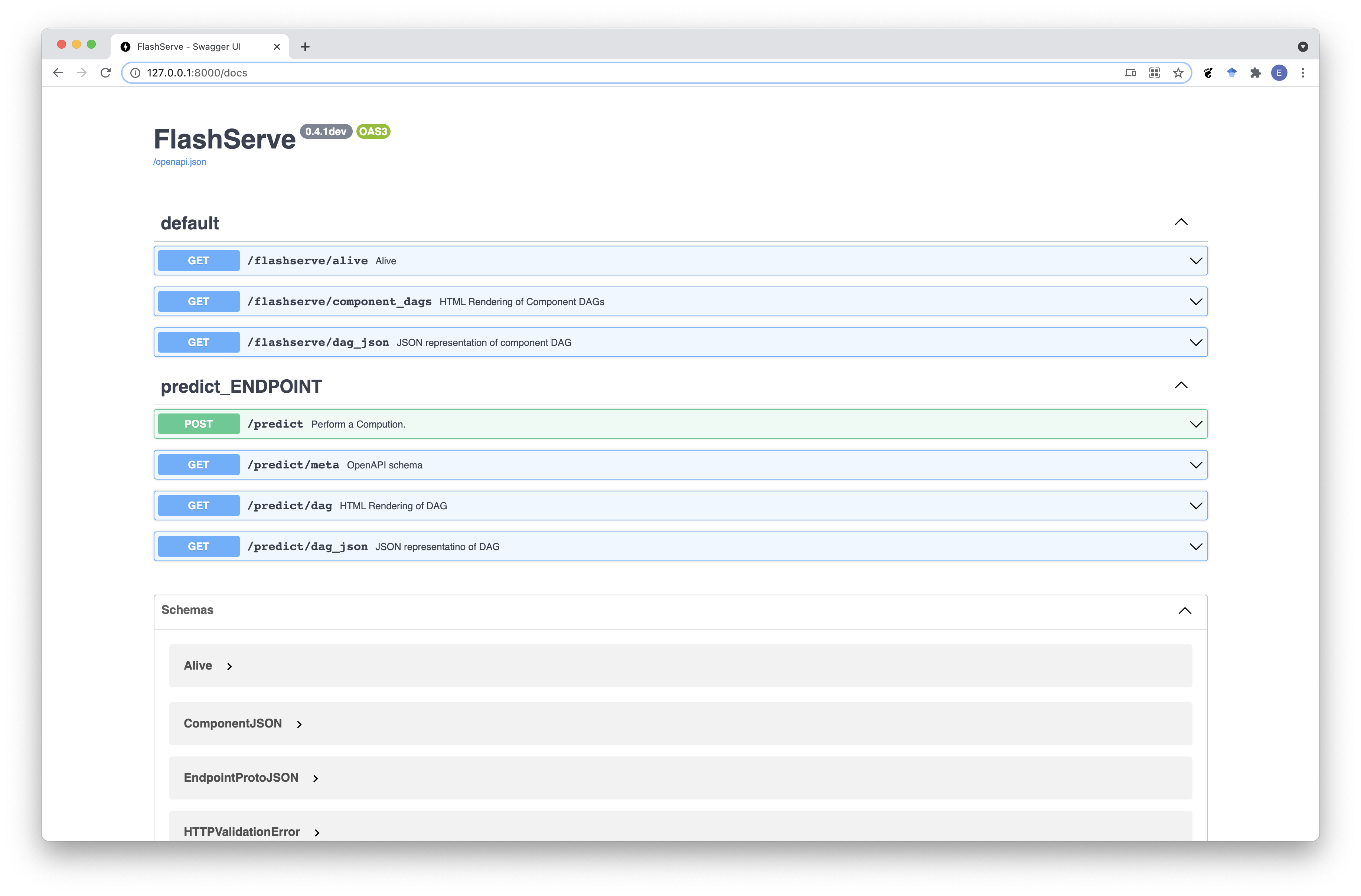Shortcuts

# Flash Serve¶

Flash Serve is a library to easily serve models in production.

## Terminology¶

Here are common terms you need to be familiar with:

Terminology

Term

Definition

de-serialization

Transform data encoded as text into tensors

inference function

A function taking the decoded tensors and forward them through the model to produce predictions.

serialization

Transform the predictions tensors back to a text encoding.

ModelComponent

The ModelComponent contains the de-serialization, inference and serialization functions.

Servable

The Servable is an helper track the asset file related to a model

Composition

The Composition defines the computations / endpoints to create & run

expose()

The expose() function is a python decorator used to augment the ModelComponent inference function with de-serialization, serialization.

## Example¶

In this tutorial, we will serve a Resnet18 from the PyTorchVision library in 3 steps.

The entire tutorial can be found under examples/serve/generic.

### Introduction¶

• de-serialization: Transform data encoded as text into tensors.

• inference function: A function taking the decoded tensors and forward them through the model to produce predictions.

• serialization: Transform the predictions tensors back as text.

In this example, we will implement only the inference function as Flash Serve already provides some built-in de-serialization and serialization functions with Image

### Step 1 - Create a ModelComponent¶

Inside inference_serve.py, we will implement a ClassificationInference class, which overrides ModelComponent.

First, we need make the following imports:

import torch
import torchvision

from flash.core.serve import Composition, Servable, ModelComponent, expose
from flash.core.serve.types import Image, LabelTo implement ClassificationInference, we need to implement a method responsible for inference function and decorated with the expose() function.

The name of the inference method isn’t constrained, but we will use classify as appropriate in this example.

Our classify function will take a tensor image, apply some normalization on it, and forward it through the model.

def classify(img):
img = img.float() / 255
mean = torch.tensor([[[0.485, 0.456, 0.406]]]).float()
std = torch.tensor([[[0.229, 0.224, 0.225]]]).float()
img = (img - mean) / std
img = img.permute(0, 3, 2, 1)
out = self.model(img)
return out.argmax()


The expose() is a python decorator extending the decorated function with the de-serialization, serialization steps.

Note

Flash Serve was designed this way to enable several models to be chained together by removing the decorator.

The expose() function takes 2 arguments:

• inputs: Dictionary mapping the decorated function inputs to BaseType objects.

• outputs: Dictionary mapping the decorated function outputs to BaseType objects.

A BaseType is a python dataclass which implements a serialize and deserialize function.

Note

Flash Serve has already several BaseType built-in such as Image or Text.

class ClassificationInference(ModelComponent):
def __init__(self, model: Servable):
self.model = model

@expose(
inputs={"img": Image()},
outputs={"prediction": Label(path="imagenet_labels.txt")},
)
def classify(self, img):
img = img.float() / 255
mean = torch.tensor([[[0.485, 0.456, 0.406]]]).float()
std = torch.tensor([[[0.229, 0.224, 0.225]]]).float()
img = (img - mean) / std
img = img.permute(0, 3, 2, 1)
out = self.model(img)
return out.argmax()


### Step 2 - Create a scripted Model¶

Using the PyTorchVision library, we create a resnet18 and use torch.jit.script to script the model.

Note

TorchScript is a way to create serializable and optimizable models from PyTorch code. Any TorchScript program can be saved from a Python process and loaded in a process where there is no Python dependency.

model = torchvision.models.resnet18(pretrained=True).eval()
torch.jit.script(model).save("resnet.pt")


### Step 3 - Serve the model¶

The Servable takes as argument the path to the TorchScripted model and then will be passed to our ClassificationInference class.

The ClassificationInference instance will be passed as argument to a Composition class.

Once the Composition class is instantiated, just call its serve() method.

resnet = Servable("resnet.pt")
comp = ClassificationInference(resnet)
composition = Composition(classification=comp)
composition.serve()


### Launching the server.¶

#### In Terminal 1¶

Just run:

python inference_server.py


And you should see this in your terminalYou should also see an Swagger UI already built for you at http://127.0.0.1:8000/docs#### In Terminal 2¶

Run this script from another terminal:

import base64
from pathlib import Path

import requests

with Path("fish.jpg").open("rb") as f:

body = {"session": "UUID", "payload": {"img": {"data": imgstr}}}
resp = requests.post("http://127.0.0.1:8000/predict", json=body)
print(resp.json())
# {'session': 'UUID', 'result': {'prediction': 'goldfish, Carassius auratus'}}


Credits to @rlizzo, @hhsecond, @lantiga, @luiscape for building Flash Serve Engine.

© Copyright 2020-2021, PyTorch Lightning. Revision a0e4620f.

Built with Sphinx using a theme provided by Read the Docs.
Versions
latest
stable
0.8.2
0.8.1.post0
0.8.1
0.8.0
0.7.5
0.7.4
0.7.3
0.7.2
0.7.1
0.7.0
0.6.0
0.5.2
0.5.1
0.5.0
0.4.0
0.3.2
0.3.1
0.3.0
0.2.3
0.2.2
0.2.1
0.2.0
0.1.0post1